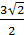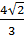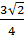### Sample Problem

Given that sec θ=3 and sin θ>0, evaluate the six trigonometric functions.

cos θ=

1/3

-1/3

3sin θ=

1/3tan θ=

√2/4

1/√2

2√2

2

cot θ=

2

√2/4

√2/2

2√2

csc θ=

6√2#### Solution

θ is in Quadrant 1 (sin θ>0 and cos θ>0)

cos θ=1/sec θ=1/3

sin θ==tan θ=cot θ=1/tan θ=1/2√2=√ 2/4

csc θ=1/sin θ=1/(2√2/3)=3/(2√2)=